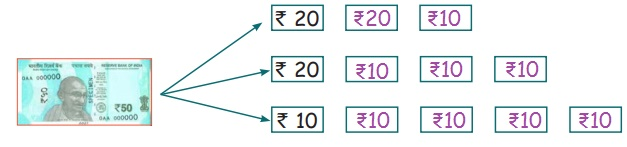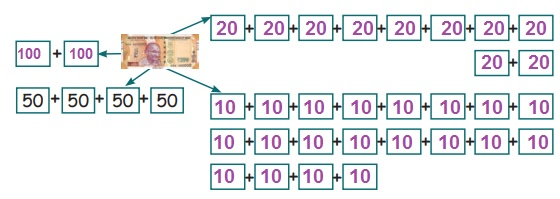Home | | Maths 4th Std | Convert rupees to paise

# Convert rupees to paise

(i) Conversion of rupees into pasie (ii) Conversion of paise into rupees

UNIT−5

MONEYConvert rupees to paise:

Our government of India indicates money as the symbol of .

Sankar is preparing to go for an Excursion. He asks his father  400 as his pocket money. Father sees that  2000 notes only in the purse. Soon father goes to shop to get change for  2000.

Father : Sir, (I need change for  2000) give me change for  2000.

Merchant : I have following varieties of combination which are as follows:Which one do you prefer?

Father : Ok sir. I select the third variety of combination. That isMerchant : Ok sir. I will give you the same.

Father : Thank you sir.

Father : Sankar, here is your  400, get it.

Sankar : Thanks pa, I need change for  100.

Father : How it would be, whether  10 note or 20 note or 50 note?Sankar : Father, shall I get the change as one  50 note, one  20 note, two  10 notes, one  5 note and 5  1 coins.Father : Ok.

Do you know?

Our fore father had used various kinds of coins. Among them 1 paise, 2 paise, 5 paise, 10 paise, 20 paise, 25 paise, 50 paise. But they are not in use now.

Denomination

2865 =  2000  500  200  100  50 10 52865

2000 × 1 = 2000

500 × 1 = 500

200 × 1 = 200

100 × 1 = 100

50 × 1 = 50

10 × 1 = 10

5 × 1 = 5

Total  2865

565 = 100 100 100 100 100 65

200 x 2 = 400

100 x 1 = 100

50 x 1 = 50

10 x 1 = 10

5 x 1 = 5

Total = 565

Try ThisActivity

Fill in the box with model notes.Activity

Take a sheet of paper and cut out the model notes ( 10,  20, and  100). Affix it below the boxes provided to get the total of  200. One is done for you.Have you ever gone to petrol bunk?

The price of 1 litre petrol is  78.12. Have you ever notice?

Although paise is not in circulation in the form of currency, but the cost of goods in paise are in use.

1 = 100 paise

Conversion of rupees into pasie.

This conversion is useful while you are bargaining in the vegetable shop and so on.

To convert rupees into paise, multiply the rupees by 100.

EXAMPLE 1

Convert  2 into paise

1 = 100 paise

So  2 = 2 x 100 = 200 paise

EXAMPLE 2

Convert  8.50 into paise

1 = 100 paise

8 is in rupees and 50 is in paise.

So we multiply by  8 with 100 and add 50 paise.

8.50 = 8 × 100 + 50

= 800 paise + 50 paise

= 850 paise

EXAMPLE 3

Ravi's father went to the shop and bought 1 kg of dhal for  38.70. Convert the cost of dhal into paise.

Cost of 1 kg dhal =  38.70

38.70 = 38 × 100 paise + 70 paise

= 3800 paise + 70 paise

Cost of dhal = 3870 paise.

Conversion of paise into rupees,

To convert paise into rupees, divide the paise by 100.

EXAMPLE 1

Convert 900 paise into rupees

To change the paise into rupees, divide the paise by 100.

900 paise = 900 ÷ 100

900 paise =  9

EXAMPLE 2

Convert 1950 paise into rupees

To change the paise into rupee, divide the paise by 100.

1950 paise = 1950 ÷ 100

= 19.50

=  19.50

Tags : Money | Term 3 Chapter 5 | 4th Maths , 4th Maths : Term 3 Unit 5 : Money
Study Material, Lecturing Notes, Assignment, Reference, Wiki description explanation, brief detail
4th Maths : Term 3 Unit 5 : Money : Convert rupees to paise | Money | Term 3 Chapter 5 | 4th Maths